Question

# Order the following functions by asymptotic growth rate: 4n, 2^log(n), 4nlog(n)+2n, 2^10, 3n+100log(n), 2^n, n^2+10n, n^3,...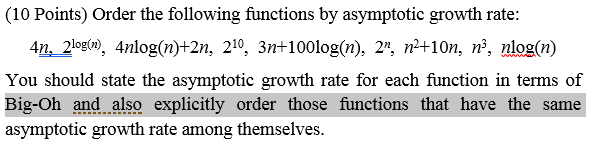Order the following functions by asymptotic growth rate: 4n, 2^log(n), 4nlog(n)+2n, 2^10, 3n+100log(n), 2^n, n^2+10n, n^3, nlog(n) You should state the asymptotic growth rate for each function in terms of Big-Oh and also explicitly order those functions that have the same asymptotic growth rate among themselves.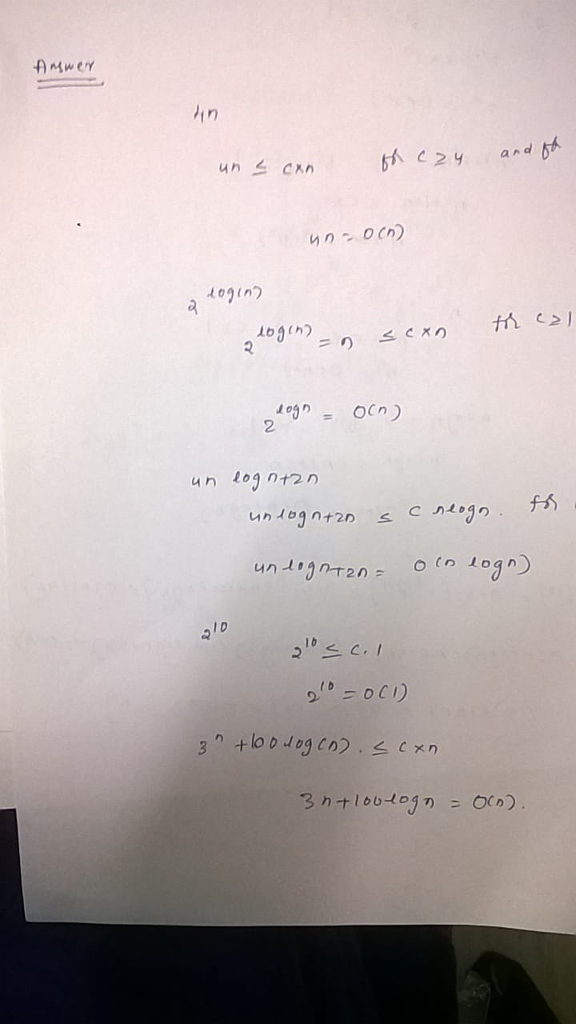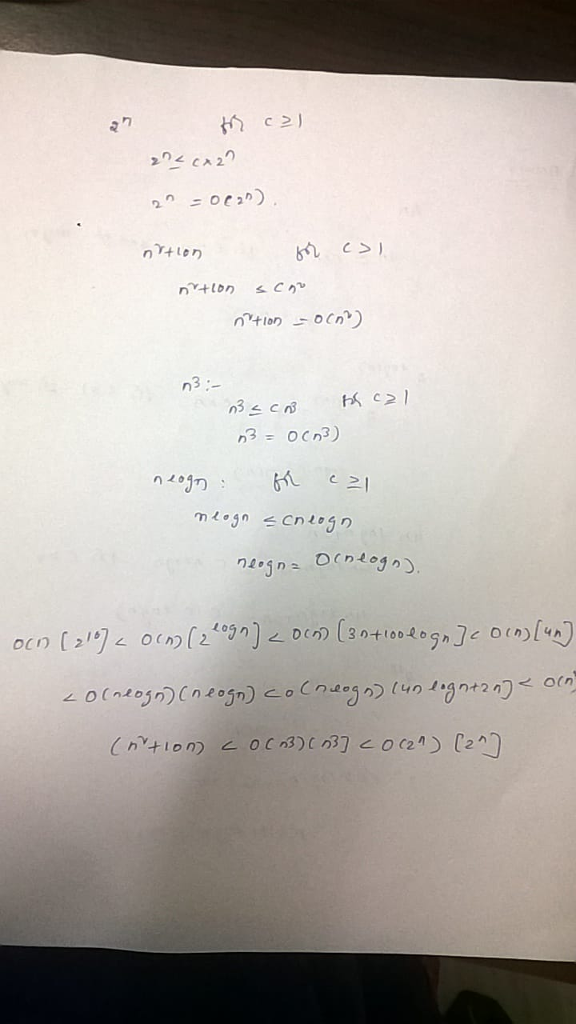#### Earn Coins

Coins can be redeemed for fabulous gifts.

Similar Homework Help Questions
• ### Order the following functions by asymptotic growth rate. 2n log n + 2n, 210, 2 log...

Order the following functions by asymptotic growth rate. 2n log n + 2n, 210, 2 log n, 3n + 100 log n, 4n, 2n, n2 + 10n, n3, n log n2

• ### Order the following functions by growth rate: N, squrerootN, N1.5, N2, NlogN, N log logN, Nlog2N,...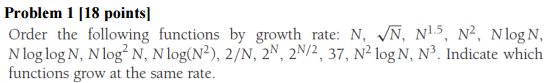Order the following functions by growth rate: N, squrerootN, N1.5, N2, NlogN, N log logN, Nlog2N, Nlog(N2), 2/N,2N, 2N/2, 37, N2 logN, N3. Indicate which functions grow at the same rate.

• ### 8.         R-4.8 Order the following functions by asymptotic growth rate. 4nlogn + 2n 2^10    2^logn 3n...

8.         R-4.8 Order the following functions by asymptotic growth rate. 4nlogn + 2n 2^10    2^logn 3n + 100logn      4n     2^n n^2 + 10n        n^3       nlogn 9.         R-4.9 Give a big-Oh characterization, in terms of n, of the running time of the example 1 method shown in Code Fragment 4.12. 10.       R-4.10 Give a big-Oh characterization, in terms of n, of the running time of the example 2 method shown in Code Fragment 4.12. 11.       R-4.11 Give a big-Oh characterization, in...

• ### 76. Arrange the following functions in ascending or- der of growth rate: 4000 log n, 2n2...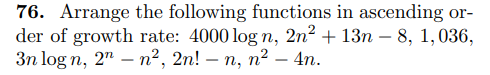76. Arrange the following functions in ascending or- der of growth rate: 4000 log n, 2n2 + 13n - 8, 1,036, 3n log n, 2" - n2, 2n! - n, n2 – 4n.

• ### Arrange the following functions in ascending order of asymptotic growth rate; that is if function g(n)...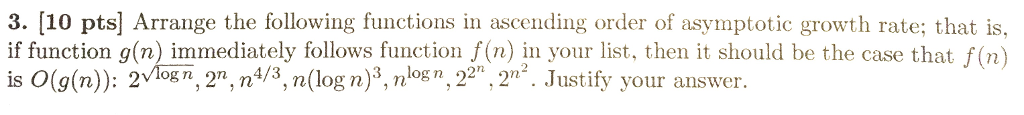Arrange the following functions in ascending order of asymptotic growth rate; that is if function g(n) immediately follows function f(n) in your list, then it should be the case that f(n) is O(g(n)): 2 Squareroot log n, 2^n, n^4/3, n(log n)^3, n log n, 2 2^n, 2^n^2. Justify your answer.

• ### Need help with 1,2,3 thank you. 1. Order of growth (20 points) Order the following functions...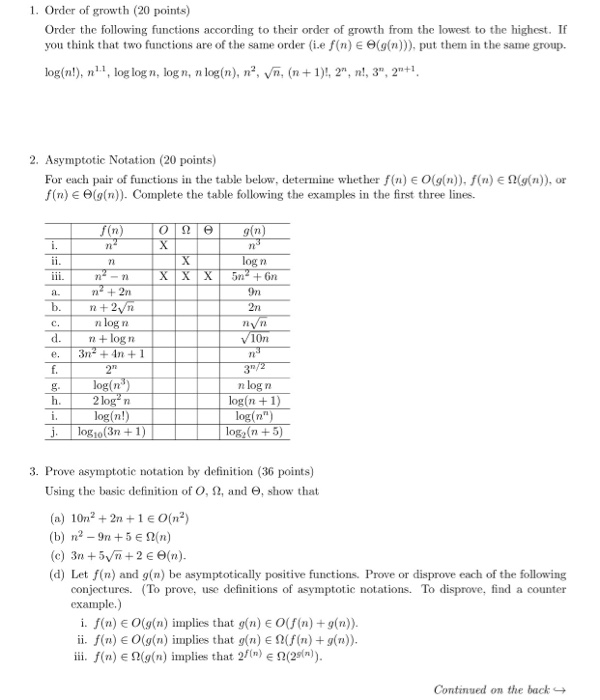Need help with 1,2,3 thank you. 1. Order of growth (20 points) Order the following functions according to their order of growth from the lowest to the highest. If you think that two functions are of the same order (Le f(n) E Θ(g(n))), put then in the same group. log(n!), n., log log n, logn, n log(n), n2 V, (1)!, 2", n!, 3", 21 2. Asymptotic Notation (20 points) For each pair of functions in the table below, deternme whether...

• ### Compare the asymptotic orders of growth of the following pairs of functions. log2 n and ....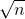Compare the asymptotic orders of growth of the following pairs of functions. log2 n and . n (n+1)/2 and n2. 2n and 3n

• ### Use the definition of 0 to show that 5n^5 +4n^4 + 3n^3 + 2n^2 + n...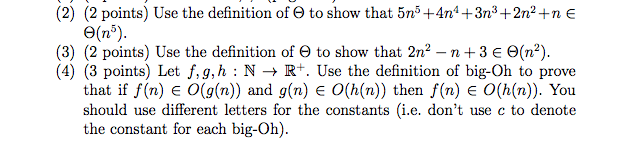Use the definition of 0 to show that 5n^5 +4n^4 + 3n^3 + 2n^2 + n 0(n^5).Use the definition of 0 to show that 2n^2 - n+ 3 0(n^2).Let f,g,h : N 1R*. Use the definition of big-Oh to prove that if/(n) 6 0(g{n)) and g(n) 0(h{n)) then/(n) 0(/i(n)). You should use different letters for the constants (i.e. don't use c to denote the constant for each big-Oh).

• ### Consider the following nine functions for the questions that follow: 1. (n^2)/2 + 3 2. 3n^3...

Consider the following nine functions for the questions that follow: 1. (n^2)/2 + 3 2. 3n^3 3. 2^n 4. 5n 5. 12n 6. 4^n 7. log_2(n) 8. log_3(n) 9. log_2(2n) (a) Make a table in which each function is in a column dictated by its big-Θ growth rate. Functions with the same asymptotic growth rate should be in the same column. If functions in one column are little-o (o(n) = O(n) - Θ(n)) of another column, put the slower growing...

• ### Order of Growth Rate Order the following functions by asymptotic growth: (i) fi(n) 3" (ii) f2(n)...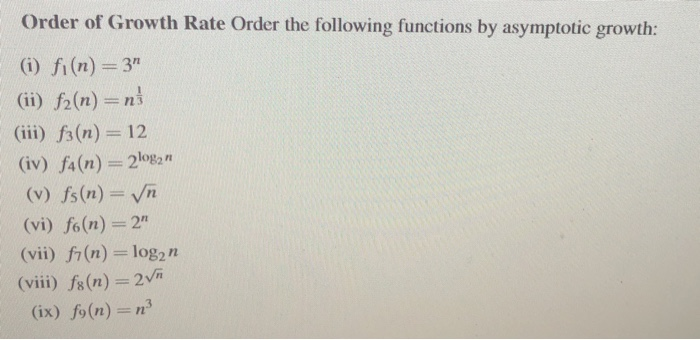Order of Growth Rate Order the following functions by asymptotic growth: (i) fi(n) 3" (ii) f2(n) ni (iii) fa(n) 12 (iv) fa(n) 2log2 n (v) fs(n) Vn (vi) f6(n) 2" (vii) fr(n) log2 n (viii) fs(n) 2V (ix) fo(n) n3